Speed of sound

The speed of sound (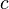$c$) can be written as: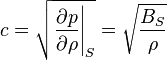$c = \sqrt{ \left. \frac{\partial p}{\partial \rho} \right\vert_S } = \sqrt{ \frac{B_S}{\rho} }$

where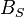$B_S$ is the adiabatic bulk modulus, given by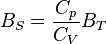$B_S = \frac{C_p}{C_V} B_T$

where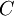$C$ is the heat capacity and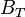$B_T$ is the isothermal bulk modulus, leading to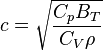$c = \sqrt{ \frac{C_p B_T}{C_V \rho} }$